# Diffusivity Equation

From Mass conservation: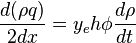$\frac{d(\rho q)}{2 dx}=y_e h \phi \frac{d\rho}{dt}$ ( 1 )

From Darcy's law: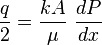$\frac{q}{2}=\frac{kA}{\mu}\ \frac{dP}{dx}$ ( 2 )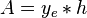$A =y_e*h$

( 2 ) →( 1 ):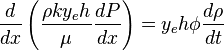$\frac{d}{dx} \left ( \frac{\rho k y_e h}{\mu} \frac{dP}{dx} \right )=y_e h \phi \frac{d\rho}{dt}$ ( 3 )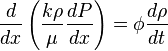$\frac{d}{dx} \left ( \frac{k \rho}{\mu} \frac{dP}{dx} \right )=\phi \frac{d\rho}{dt}$ ( 4 )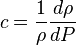$c=\frac{1}{\rho} \frac{d \rho}{dP}$ ( 5 )

( 5 ) -> ( 4 ):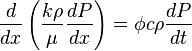$\frac{d}{dx} \left ( \frac{k \rho}{\mu} \frac{dP}{dx} \right )=\phi c \rho \frac{dP}{dt}$ ( 6 )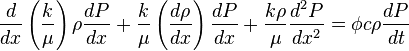$\frac{d}{dx} \left ( \frac{k}{\mu} \right ) \rho \frac{dP}{dx} + \frac{k}{\mu} \left ( \frac{d \rho}{dx} \right ) \frac{dP}{dx} + \frac{k \rho}{\mu} \frac{d^2P}{dx^2}=\phi c \rho \frac{dP}{dt}$ ( 7 )

Assumption that viscosity is constant cancels out first term in left hand side of (7):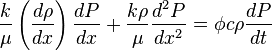$\frac{k}{\mu} \left ( \frac{d \rho}{dx} \right ) \frac{dP}{dx} + \frac{k \rho}{\mu} \frac{d^2P}{dx^2}=\phi c \rho \frac{dP}{dt}$ ( 8 )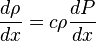$\frac{d \rho}{dx} = c \rho \frac{d P}{dx}$ ( 9 )

( 9 ) -> ( 8 ):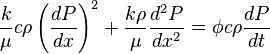$\frac{k}{\mu} c \rho \left ( \frac{dP}{dx} \right )^2+ \frac{k \rho}{\mu} \frac{d^2P}{dx^2}=\phi c \rho \frac{dP}{dt}$ ( 10 )

Term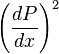$\left ( \frac{dP}{dx} \right )^2$ in (10) is second order of magnitude low and can be cancelled out, which yields: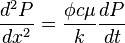$\frac{d^2P}{dx^2}=\frac{\phi c \mu}{k} \frac{dP}{dt}$ ( 11 )